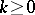# Weil algebra of a Lie algebra

(diff) ← Older revision | Latest revision (diff) | Newer revision → (diff)

Let $G$ be a connected Lie group with Lie algebra $\frak g$. The Weil algebra $W ( \mathfrak{g} )$ of $\frak g$ was first introduced in a series of seminars by H. Cartan [a1], in part based on some unpublished work of A. Weil. As a differential graded algebra, it is given by the tensor product

\begin{equation*} W ( \mathfrak { g } ) = \bigwedge \mathfrak { g } ^ { * } \bigotimes S \mathfrak { g } ^ { * }, \end{equation*}

where $\wedge \mathfrak { g } ^ { * }$ and $S \mathfrak { g } ^ { * }$ denote the exterior and symmetric algebras, respectively (cf. also Exterior algebra; Symmetric algebra).

The Weil algebra and its generalizations have been studied extensively by F.W. Kamber and Ph. Tondeur [a3] [a4]. Let $K \subseteq G$ be a maximal compact subgroup, with $\frak p$ denoting the Lie algebra of $K$. The relative Weil algebra for $( G , K )$ is defined by

\begin{equation*} W ( G , K ) = \{ \bigwedge ( \mathfrak { g } / \mathfrak { k } ) ^ { * } \bigotimes S \mathfrak { g } ^ { * } \} ^ { K }. \end{equation*}

With regards to the universal classifying bundle $E G \rightarrow B G$ (cf. also Bundle; Classifying space; Universal space), there are canonical isomorphisms in cohomologywhere $I ( K )$ denotes the $\operatorname{Ad} K$-invariant polynomials. For a given integer, one has the ideal

\begin{equation*} F W = F ^ { 2 ( k + 1 ) } W ( G , K ) \subseteq W ( G , K ), \end{equation*}

generated by $S ^ { \text{l} } ( \mathfrak { g } ^ { * } )$, for $\text{l} \geq k + 1$. This leads to the truncated Weil algebra

\begin{equation*} W _ { k } = W ( G , K ) _ { k } = W ( G , K ) / F W. \end{equation*}

The cohomology $H ^ { * } ( W _ { k } )$ plays a prominent role in the study of secondary characteristic classes (cf. also Characteristic class) of foliations and foliated bundles [a3] (see also [a2]).

How to Cite This Entry:
Weil algebra of a Lie algebra. Encyclopedia of Mathematics. URL: http://encyclopediaofmath.org/index.php?title=Weil_algebra_of_a_Lie_algebra&oldid=50839
This article was adapted from an original article by James F. Glazebrook (originator), which appeared in Encyclopedia of Mathematics - ISBN 1402006098. See original article